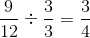SSAT Middle Level Math : How to add fractions

Example Questions

Example Question #1 : Adding Mixed Numbers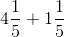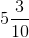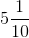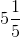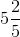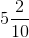Explanation:

When we add mixed numbers, we add whole numbers to whole numbers and fractions to fractions.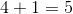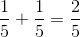Remember, when we are adding fractions we must have common denominators and we only add the numerators.

Example Question #2 : Adding Mixed Numbers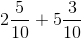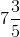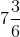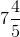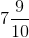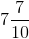Explanation:

When we add mixed numbers, we add whole numbers to whole numbers and fractions to fractions.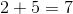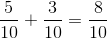Remember, when we are adding fractions we must have common denominators and we only add the numerators.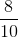can be reduced by dividingto both sides.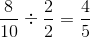Example Question #3 : Adding Mixed Numbers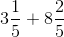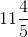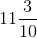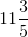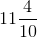Explanation:

When we add mixed numbers, we add whole numbers to whole numbers and fractions to fractions.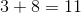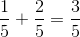Remember, when we are adding fractions we must have common denominators and we only add the numerators.

Example Question #5 : Adding Mixed Numbers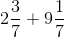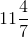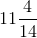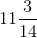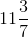Explanation:

When we add mixed numbers, we add whole numbers to whole numbers and fractions to fractions.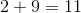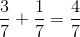Remember, when we are adding fractions we must have common denominators and we only add the numerators.

Example Question #6 : Adding Mixed Numbers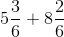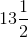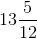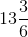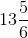Explanation:

When we add mixed numbers, we add whole numbers to whole numbers and fractions to fractions.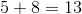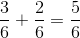Remember, when we are adding fractions we must have common denominators and we only add the numerators.

Example Question #1 : Adding Mixed Numbers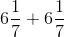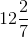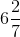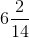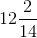Explanation:

When we add mixed numbers, we add whole numbers to whole numbers and fractions to fractions.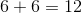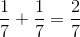Remember, when we are adding fractions we must have common denominators and we only add the numerators.

Example Question #8 : Adding Mixed Numbers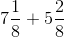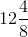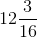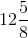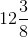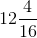Explanation:

When we add mixed numbers, we add whole numbers to whole numbers and fractions to fractions.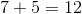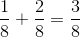Remember, when we are adding fractions we must have common denominators and we only add the numerators.

Example Question #514 : Number & Operations: Fractions

Solve: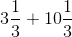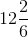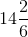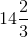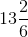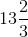Explanation:

When we add mixed numbers, we add whole numbers to whole numbers and fractions to fractions.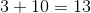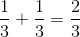Remember, when we are adding fractions we must have common denominators and we only add the numerators.

Example Question #515 : Number & Operations: Fractions

Solve: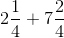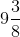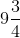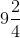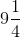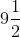Explanation:

When we add mixed numbers, we add whole numbers to whole numbers and fractions to fractions.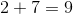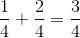Remember, when we are adding fractions we must have common denominators and we only add the numerators.

Example Question #21 : Solve Word Problems Involving Addition And Subtraction Of Fractions: Ccss.Math.Content.5.Nf.A.2

Sally drank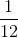of the milk and Sam drank. What fraction of the milk did they drink?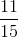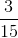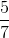Explanation: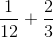In order to solve this problem, we first need to make common denominators.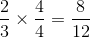Now that we have common denominators, we can add the fractions. Remember, when we add fractions, the denominator stays the same, we only add the numerator.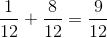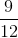can be reduced by dividingby both sides.